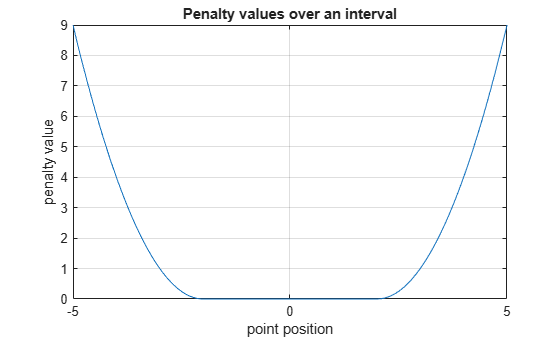exteriorPenalty

Exterior penalty value for a point with respect to a bounded region

Description

example

p = exteriorPenalty(x,xmin,xmax,method) uses the specified method to calculate the nonnegative (exterior) penalty vector p for the point x with respect to the region bounded by xmin and xmax. p has the same dimension as x.

Examples

collapse all

This example shows how to use the exteriorPenalty function to calculate the exterior penalty for a given point, with respect to a bounded region.

Calculate the penalty value for the point 0.1 within the interval [-2,2], using the step method.

exteriorPenalty(0.1,-2,2,'step')
ans = 0

Calculate the penalty value for the point 4 outside the interval [-2,2], using the step method.

exteriorPenalty(4,-2,2,'step')
ans = 1

Calculate the penalty value for the point 4 outside the interval [-2,2], using the quadratic method.

ans = 4

Calculate the penalty value for the point [-2,0,4] with respect to the box defined by the intervals [0,1], [-1,1], and [-2,2] along the x, y, and z dimensions, respectively, using the quadratic method.

exteriorPenalty([-2 0 4],[0 -1 -2],[1 1 2],'quadratic')
ans = 3×1

4
0
4

Visualize Penalty Values for an Interval

Create a vector of 1001 equidistant points distributed between -5 and 5.

x = -5:0.01:5;

Calculate penalties for all the points in the vector, using the quadratic method.

Plot the points and add grid, labels, and title.

plot(x,p)
grid
xlabel("point position");
ylabel("penalty value");
title("Penalty values over an interval");Input Arguments

collapse all

Point for which the exterior penalty is calculated, specified as a numeric scalar, vector, or matrix.

Example: [-0.1, 1.3]

Lower bounds for x, specified as a numeric scalar, vector, or matrix. To use the same minimum value for all elements in x, specify xmin as a scalar.

Example: -2

Upper bounds for x, specified as a numeric scalar, vector, or matrix. To use the same maximum value for all elements in x, specify xmax as a scalar.

Example: [5 10]

Function used to calculate the penalty, specified either as 'step' or 'quadratic'. You can also use strings instead of character vectors.

Output Arguments

collapse all

Penalty value, returned as a vector of nonnegative elements. With either of the two methods, each element pi is zero if the corresponding xi is within the region specified by xmini and xmaxi, and it is positive otherwise. Penalty functions are typically used to generate negative rewards when constraints are violated, such as in generateRewardFunction.